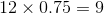# ISEE Middle Level Quantitative : Percentage

## Example Questions

← Previous 1 3 4 5 6 7 8

### Example Question #1 : Percentage

Using the information given in each question, compare the quantity in Column A to the quantity in Column B.

Column A                       Column B

120% of 80% of 100       130% of 70% of 105

The quantity in Column A is greater.

The two quantities are equal.

The quantity in Column B is greater.

The relationship cannot be determined from the information given.

The quantity in Column A is greater.

Explanation:

Remember that "percent" means "per 100" and "of" means to multiply.

So 120% of 80% of 100 =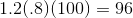and 130% of 70% of 105 =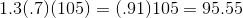Column A is greater.

### Example Question #2 : Percentage

Which is the greater quantity?

(a)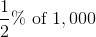(b)(a) and (b) are equal

(b) is greater

It is impossible to tell from the information given

(a) is greater

(a) is greater

Explanation:can be rewritten as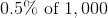, and restated as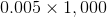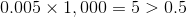### Example Question #3 : Percentage

What is 60% of 60% of 25,000?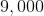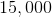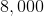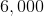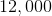Explanation:

60% of 25,000 is equal to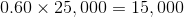60% of that is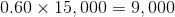### Example Question #4 : Percentageis a positive number. Which of the following is the greater quantity?

(A) 50% of(B) 20% of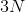(B) is greater

(A) is greater

It is impossible to determine which is greater from the information given

(A) and (B) are equal

(B) is greater

Explanation:

50% ofis equal to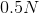.

20% ofis equal to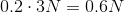Sinceis positive and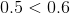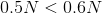,

and (B) is greater.

### Example Question #5 : Percentage

What is 42 percent of 5?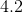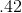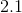Explanation:

The easiest way to find 42 percent of 5 is to first find 42 percent of 10.

42 percent of 10 can be calculated by multiplying .42 by 10. This results in 4.2.

Given that 5 is half of 10, it follows that 42 percent of 5 is equal to half of 4.2.

Half of 4.2 is equal to 2.1, which is the correct answer.

### Example Question #6 : Percentage

Katie collects trading cards. 15% of her collection are rare cards. If there are a total of 500 cards in Katie's collection, how many of them are rare?Explanation:

To begin, you can set up a proportion: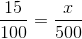When trying to find a percent of a number, convert the percent into a decimal. To do this, divide the percent by 100.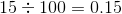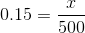You then multiply 500 by 0.15. Since 500 represents 100% of Katie's cards, multiplying by 0.15 will give you how many cards equal 15% of the total.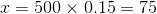### Example Question #7 : Percentage

What is 10% of 30% of 100?Explanation:

This question asks you to find a percentage of a smaller part of 100. You must first figure out what the smaller part of 100 equals. Since percents are based off of 100, 30% of 100 is the number 30. You must then find 10% of this number.

To do this, multiply 30 times 10%. First, divide the percentage by 100.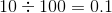Multiply the result times 30.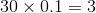### Example Question #8 : Percentage

Amir will be selling 20% of his stamp collection. If he has 60 stamps now, how many will he have after he sells some of his stamps?Explanation:

If Amir gets rid of 20% of his cards, he will only have 80% of his cards remaining afterwards.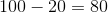Therefore, we can figure out how many cards Amir will have after selling 20% of his cards by multiplying times 80% since that will be the amount of cards he will have left, compared to the original number.

To do this, first divide the percentage by 100.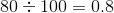Then, multiply the result of this times 60.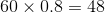### Example Question #9 : Percentage

What is 60% of 120?Explanation:

In order to figure out what 60% of 120 is, multiply 60% by 120. To do this, first divide your percentage by 100.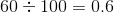Then, multiply the result times 120.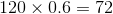### Example Question #10 : Percentage

What is 75% of 25% of 48?Explanation:

The question asks you to find 75% of a smaller part of 48. In order to figure this out, you must first figure out what the value of the smaller part is. To figure out what 25% of 48 is, multiply 48 by 25%. First, divide the percentage by 100.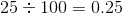Then, multiply 48 by the result.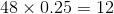The second part of the question asks you to figure out what 75% of this new number is. Just like before, first divide the percentage by 100.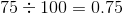Then, multiply the result times 12.